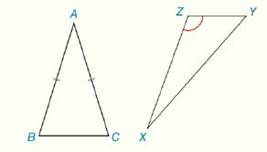Chapter 4.CR, Problem 35CRElementary Geometry For College St...

7th Edition
Alexander + 2 others
ISBN: 9781337614085

Solutions

Chapter
SectionElementary Geometry For College St...

7th Edition
Alexander + 2 others
ISBN: 9781337614085
Textbook Problem

Review Exercises What type of quadrilateral is formed when the triangle is reflected across the indicated side? a) Isosceles ∆ A B C across B C - b) Obtuse ∆ X Y Z across X Y -To determine

To Find:

The type of quadrilateral is formed when the isosceles ΔABC across BC¯.

Explanation

Definition:

A rhombus is a parallelogram with two congruent adjacent sides.

Properties:

1. All sides of a rhombus are congruent.

2. The diagonals of a rhombus are perpendicular.

3. The diagonals of a rhombus are perpendicular bisector of each other.

Definition:

A kite is a quadrilateral with two distinct pairs of congruent adjacent sides...

To determine

To Find:

The type of quadrilateral is formed when the Obtuse ΔXYZ across XY¯.

Still sussing out bartleby?

Check out a sample textbook solution.

See a sample solution

The Solution to Your Study Problems

Bartleby provides explanations to thousands of textbook problems written by our experts, many with advanced degrees!

Get Started

Solve the equations in Exercises 112 for x (mentally, if possible). x3=1

Finite Mathematics and Applied Calculus (MindTap Course List)

In Exercises 63-68, use the graph of the function f to determine limxf(x) and limxf(x) 66.

Applied Calculus for the Managerial, Life, and Social Sciences: A Brief Approach

Evaluate dy if y = x3 2x2 + 1, x = 2, and dx = 0.2.

Single Variable Calculus: Early Transcendentals

Which vector at the right could be a gradient for f(x, y)? a b c d

Study Guide for Stewart's Multivariable Calculus, 8th